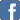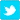# Mole Concept Questions on Excess and Limiting Reagent

Posted on the 15 January 2022 by Ewong0117

In this post, let's try a question on excess and limiting reagent. This type of questions come from the chapter mole concept and stoichiometry.

Question

1.0 mole of Cu3FeS3 and 1.0 mole of O2 are allowed to react according to the following equation:

2Cu3FeS3(s) + 7O2(g)  --> 6Cu (s) + 2FeO(s) + 6SO2 (g)

Which of the following statement is true?

A. 0.286 mol of Cu3FeS3 is in excess

B. 0.714 mol of Cu3FeS3 is in excess

C. 5.0 mol of O2 is in excess

D. no reagent is in excess

Explanation

From the equation, mole ratio of Cu3FeS3:O is 2:7.

From the above equation, we know that we need more (moles) of  Cu3FeS3 than O. Since we use the same amount of both, but we need more O2 for the reaction, this means Cu3FeSis in excess (or O2 is the limiting reagent). Hence, options C and D are incorrect.

Now, we know is in excess, hence, all of it will react as it is the limiting reagent.

2 mol of  Cu3FeS3: 7 mol of:O

2/7  mol of  Cu3FeS3: 1 mol of:O

Since 1 mol of Cu3FeS3 is used,  1 - 2/7 = 5/7 mol of Cu3FeSis in excess.

5/7 = 0.714 mol (3 decimal places)

Hence, 0.714 mol of Cu3FeS3 is in excess. The correct answer option is B.